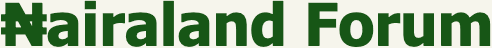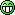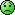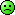#Welcome, Guest: Join Nairaland / LOGIN! / Trending / Recent / New
Stats: 2,523,959 members, 5,774,574 topics. Date: Thursday, 06 August 2020 at 11:44 PM

## My Collections Of Python Projects - Programming - Nairaland

(1) (2) (3) (Reply) (Go Down)

 My Collections Of Python Projects by KlausMichaelson: 11:13pm On Jul 29 I am so glad that this journey have started yielding good fruits. My dream is to become an expert in Python one day and teach people how to write the language like Mosh Hamedani, Cs Dojo and Hamit Ranjit Grom ACADGILDThis is making it a month of Learning Python. Although I've seen some videos on python long before now but I didn't take it too serious. Already I have interest in Data Analysis but I still have some feelings towards Software development but that will be later on when I'm done learning Data Science and mastering all the tools in it.So I'm going to be Posting some codes I write by myself everyday so as to keep the fire Burning. Please I will welcome any correction gladly. Thanks be to God for the Holidays. This is ironically a bad year indeed.Modified:Please you can also give me a task or exercise to Work on. I'll be grateful. 1 Like Re: My Collections Of Python Projects by KlausMichaelson: 11:16pm On Jul 29 So I spent the previous night thinking of writing a code that can accept input from a user and run different operations on it. The operations are limited to Addition, subtraction, Division, Multiplication, and Modulo. I came up with this after much thought. number1 = int(input('what number? '))number2 = int(input('what number? '))calculation = input('what operation do you want? ')def operation(num1,num2): addition = num1 + num2 subtraction = num1 - num2 multiplication = num1 * num2 division = num1 / num2 modulo = num1 % num2 if calculation == 'addition': return addition elif calculation == 'subtraction': return subtraction elif calculation == 'multiplication': return multiplication elif calculation == "division": return division elif calculation == 'modulo': return modulo result = operation(number1, number2)print(result)Modified:After taking Corrections from my Top Ogas in the House, I finally came up with a better code for the calculator.Corrections are still welcomed.number1 = int(input('Enter a number? '))number2 = int(input('Enter another number? '))calculation = input('addition, subtraction, multiplication, or division? ').lower()def operation(num1, num2): if calculation == 'addition': return num1 + num2 elif calculation == 'subtraction': return num1 - num2 elif calculation == 'multiplication': return num1 * num2 elif calculation == "division": try: if num1 % num2 == 0: return num1 / num2 else: return num1/num2 except ZeroDivisionError: print('cannot divide zero, enter a denominator other than zero')result = operation(number1, number2)print(result) Re: My Collections Of Python Projects by KlausMichaelson: 11:18pm On Jul 29 Please I'll be very happy if anyone could give me a task to complete. I would try to do it. Thanks a lot. Re: My Collections Of Python Projects by Akhigbeblog(m): 11:37pm On Jul 29 KlausMichaelson:Please I'll be very happy if anyone could give me a task to complete. I would try to do it. Thanks a lot.How did you get the videos you have?? Re: My Collections Of Python Projects by KlausMichaelson: 11:46pm On Jul 29 Akhigbeblog: How did you get the videos you have?? I got some through a course mate and others were from YouTube. Re: My Collections Of Python Projects by Donotread: 11:52pm On Jul 29 KlausMichaelson:Please I'll be very happy if anyone could give me a task to complete. I would try to do it. Thanks a lot.What type of Task Re: My Collections Of Python Projects by KlausMichaelson: 11:56pm On Jul 29 Donotread:What type of TaskSir any task at all. It could be to write a code to perform a particular function, I'll be very willing to do it. Re: My Collections Of Python Projects by Donotread: 11:57pm On Jul 29 KlausMichaelson:Sir any task at all. It could be to write a code to perform a particular function, I'll be very willing to do it.To learn Re: My Collections Of Python Projects by KlausMichaelson: 11:59pm On Jul 29 Donotread:To learnOfcourse at the same time I'll learn. If I attempt it and I fail to get it, you may voluntarily give me the correct code for the task Sir. Re: My Collections Of Python Projects by Donotread: 12:01am On Jul 30 KlausMichaelson:Ofcourse at the same time I'll learn. If I attempt it and I fail to get it, you may voluntarily give me the correct code for the task Sir.OK Re: My Collections Of Python Projects by KlausMichaelson: 12:03am On Jul 30 Donotread:OK Alright and thank you Sir Re: My Collections Of Python Projects by fortifiedng: 1:14am On Jul 30 Why dont you visit edabit.com, you'd get enough coding challenges there. 1 Like Re: My Collections Of Python Projects by Darivie04(m): 6:23am On Jul 30 KlausMichaelson:So I spent the previous night thinking of writing a code that can accept input from a user and run different operations on it. The operations are limited to Addition, subtraction, Division, Multiplication, and Modulo. I came up with this after much thought. number1 = int(input('what number? '))number2 = int(input('what number? '))calculation = input('what operation do you want? ')def operation(num1,num2): addition = num1 + num2 subtraction = num1 - num2 multiplication = num1 * num2 division = num1 / num2 modulo = num1 % num2 if calculation == 'addition': return addition elif calculation == 'subtraction': return subtraction elif calculation == 'multiplication': return multiplication elif calculation == "division": return division elif calculation == 'modulo': return modulo result = operation(number1, number2)print(result)number1 = int(input('what number? '))number2 = int(input('what number? '))calculation = input('what operation do you want? ')def operation(num1,num2): if calculation == 'addition': return num1 + num2 elif calculation == 'subtraction': return num1 - num2 elif calculation == 'multiplication': return num1 * num2 elif calculation == "division": return num1 / num2 elif calculation == 'modulo': return num1 % num2 print (operation(number1, number2))A bit neater. 2 Likes 1 Share Re: My Collections Of Python Projects by KlausMichaelson: 6:47am On Jul 30 fortifiedng:Why dont you visit edabit.com, you'd get enough coding challenges there. I'll check it out sir. Thank you Re: My Collections Of Python Projects by KlausMichaelson: 6:49am On Jul 30 Darivie04: number1 = int(input('what number? ')) number2 = int(input('what number? ')) calculation = input('what operation do you want? ') def operation(num1,num2): if calculation == 'addition': return num1 + num2 elif calculation == 'subtraction': return num1 - num2 elif calculation == 'multiplication': return num1 * num2 elif calculation == "division": return num1 / num2 elif calculation == 'modulo': return num1 % num2 print (operation(number1, number2)) A bit neater. Honestly this is Superbthank you so much. Re: My Collections Of Python Projects by Akhigbeblog(m): 7:18am On Jul 30 KlausMichaelson: I got some through a course mate and others were from YouTube.I need offline videosRe: My Collections Of Python Projects by KlausMichaelson: 8:43am On Jul 30 Darivie04:number1 = int(input('what number? '))number2 = int(input('what number? '))calculation = input('what operation do you want? ')def operation(num1,num2): if calculation == 'addition': return num1 + num2 elif calculation == 'subtraction': return num1 - num2 elif calculation == 'multiplication': return num1 * num2 elif calculation == "division": return num1 / num2 elif calculation == 'modulo': return num1 % num2 print (operation(number1, number2))A bit neater.Sir how long have you been learning the language??And what category would you place yourself?? Re: My Collections Of Python Projects by KlausMichaelson: 8:44am On Jul 30 Akhigbeblog:I need offline videosWhere do you reside?? Re: My Collections Of Python Projects by ibromodzi: 10:12am On Jul 30 KlausMichaelson:So I spent the previous night thinking of writing a code that can accept input from a user and run different operations on it. The operations are limited to Addition, subtraction, Division, Multiplication, and Modulo. I came up with this after much thought. number1 = int(input('what number? '))number2 = int(input('what number? '))calculation = input('what operation do you want? ')def operation(num1,num2): addition = num1 + num2 subtraction = num1 - num2 multiplication = num1 * num2 division = num1 / num2 modulo = num1 % num2 if calculation == 'addition': return addition elif calculation == 'subtraction': return subtraction elif calculation == 'multiplication': return multiplication elif calculation == "division": return division elif calculation == 'modulo': return modulo result = operation(number1, number2)print(result)Your program assumes you'll be the only user who knows the right keywords to enter in order to get the desired result. What tells the user the kind of operations your program is capable of performing?What happens if the user does not enter the operations to be performed correctly as used in the program? Like Addition instead of addition (issue of cases).What happens if the user tries to divide a number by zero?When writing codes, I always like to think like a typical user who'll love to experiment with any possible combinations.I challenge you to solve the problems above and update your code.... Re: My Collections Of Python Projects by KlausMichaelson: 10:21am On Jul 30 ibromodzi:Your program assumes you'll be the only user who knows the right keywords to enter in order to get the desired result. What tells the user the kind of operations your program is capable of performing?What happens if the user does not enter the operations to be performed correctly as used in the program? Like Addition instead of addition (issue of cases).What happens if the user tries to divide a number by zero?When writing codes, I always like to think like a typical user who'll love to experiment with any possible combinations.I challenge you to solve the problems above and update your code....Ha SirThere is no problem Sir. I am grateful for you Assessment. Thank you.*What tells the user the kind of operations my program can run?* What happens if the user does not enter the operations to be performed correctly as used in the program? Like Addition instead of addition (issue of cases)?*What happens if the user tries to divide a number by zero?Sir, I will give the updated code for it later today. Thank you very much sir. I really appreciate. Re: My Collections Of Python Projects by ibromodzi: 10:33am On Jul 30 KlausMichaelson:Ha SirThere is no problem Sir. I am grateful for you Assessment. Thank you.*What tells the user the kind of operations my program can run?* What happens if the user does not enter the operations to be performed correctly as used in the program? Like Addition instead of addition (issue of cases)?*What happens if the user tries to divide a number by zero?Sir, I will give the updated code for it later today. Thank you very much sir. I really appreciate.Alright..... You are welcome sir.You may add an extra bonus by implementing your solution in OOP (not necessary though).Cheers! Re: My Collections Of Python Projects by Patiee: 10:55am On Jul 30 Excuse me, I'm out of the topic. Does anybody here know how long it takes for the openWeather API keys to be activated. They emailed me that it will take a couple of hours and it has been a day now Re: My Collections Of Python Projects by KlausMichaelson: 11:09am On Jul 30 ibromodzi:Alright..... You are welcome sir.You may add an extra bonus by implementing your solution in OOP (not necessary though).Cheers!Sir bit by bit. I won't promise adding the extra bonus. But I'll try..Anyways I really appreciate your responses. Thank you Re: My Collections Of Python Projects by Akhigbeblog(m): 1:18pm On Jul 30 KlausMichaelson:Where do you reside??Abuja Re: My Collections Of Python Projects by ARCHEMEDESME(m): 5:23pm On Jul 30 Darivie04: number1 = int(input('what number? ')) number2 = int(input('what number? ')) calculation = input('what operation do you want? ') def operation(num1,num2): if calculation == 'addition': return num1 + num2 elif calculation == 'subtraction': return num1 - num2 elif calculation == 'multiplication': return num1 * num2 elif calculation == "division": return num1 / num2 elif calculation == 'modulo': return num1 % num2 print (operation(number1, number2)) A bit neater. I would like to ask you a few questions on WhatsApp if you don't mind Re: My Collections Of Python Projects by KlausMichaelson: 6:31pm On Jul 30 Akhigbeblog: Abuja I wish I can help but distance is the barrier. Re: My Collections Of Python Projects by Taofeekdboy(m): 7:16pm On Jul 30 KlausMichaelson:So I spent the previous night thinking of writing a code that can accept input from a user and run different operations on it. The operations are limited to Addition, subtraction, Division, Multiplication, and Modulo. I came up with this after much thought. number1 = int(input('what number? '))number2 = int(input('what number? '))calculation = input('what operation do you want? ')def operation(num1,num2): addition = num1 + num2 subtraction = num1 - num2 multiplication = num1 * num2 division = num1 / num2 modulo = num1 % num2 if calculation == 'addition': return addition elif calculation == 'subtraction': return subtraction elif calculation == 'multiplication': return multiplication elif calculation == "division": return division elif calculation == 'modulo': return modulo result = operation(number1, number2)print(result)based on what ibromodzi has said, I could see ZeroDivisionError, TypeError and other Errors in this code,When writing mostly inputation code, you need to validate user's input because if you fail to do so, your program will break as Python is a runtime language. Try to put it in 'try' and 'exception' block.. I wish you Goodluck in your learning. Re: My Collections Of Python Projects by KlausMichaelson: 7:25pm On Jul 30 Taofeekdboy:based on what ibromodzi has said, I could see ZeroDivisionError, TypeError and other Errors in this code,When writing mostly inputation code, you need to validate user's input because if you fail to do so, your program will break as Python is a runtime language. Try to put it in 'try' and 'exception' block.. I wish you Goodluck in your learning. Thank you sir. I'm about to turn on my system. I'll send the updated code anytime soon. Re: My Collections Of Python Projects by KlausMichaelson: 8:39pm On Jul 30 ibromodzi:Your program assumes you'll be the only user who knows the right keywords to enter in order to get the desired result. What tells the user the kind of operations your program is capable of performing?What happens if the user does not enter the operations to be performed correctly as used in the program? Like Addition instead of addition (issue of cases).What happens if the user tries to divide a number by zero?When writing codes, I always like to think like a typical user who'll love to experiment with any possible combinations.I challenge you to solve the problems above and update your code....Good evening SirYour question:What tells the user the kind of operations your program is capable of performing?My Answer:Calculation = input('addition, subtraction, multiplication, division, or modulo? ')Your Question:What happens if the user does not enter the operations to be performed correctly as used in the program? Like Addition instead of addition (issue of cases).My Answer:I use .lower if I want any input to be in lower case i.e Calculation = input('addition, subtraction, multiplication, division, or modulo? ').lowerYour Question:What happens if the user tries to divide a number by zero?My Answer:You use try except ZeroDivisionError or ValueErrorBut Sir I don't know where to to put in this try, except block in my programThe reason why I am yet to update my new code after about an hour now is because I don't know the position where the try..... except ZeroDivisionError and except ValueError block comes in. Please help me sir.Thank you Re: My Collections Of Python Projects by Akhigbeblog(m): 9:36pm On Jul 30 KlausMichaelson: I wish I can help but distance is the barrier.Do you have link which I can use to to download the videos from? Re: My Collections Of Python Projects by Taofeekdboy(m): 10:14pm On Jul 30 KlausMichaelson:Good evening SirYour question:What tells the user the kind of operations your program is capable of performing?My Answer:Calculation = input('addition, subtraction, multiplication, division, or modulo? ')Your Question:What happens if the user does not enter the operations to be performed correctly as used in the program? Like Addition instead of addition (issue of cases).My Answer:I use .lower if I want any input to be in lower case i.e Calculation = input('addition, subtraction, multiplication, division, or modulo? ').lowerYour Question:What happens if the user tries to divide a number by zero?My Answer:You use try except ZeroDivisionError or ValueErrorBut Sir I don't know where to to put in this try, except block in my programThe reason why I am yet to update my new code after about an hour now is because I don't know the position where the try..... except ZeroDivisionError and except ValueError block comes in. Please help me sir.Thank youI am through for the day as it is very late here, I would have shown you the code but you have to change the logic if you want to use the try and exept block.I recommend while loop, if it's true, then it will keep requesting for the user's input if there's an error until you put a condition to meet. Read more on while loop, try and exeption. As a beginner, try to fix things on your own, when you reach that point you feel you are lost then you can Google or use stackoverflow. Goodnight. 1 Like Re: My Collections Of Python Projects by KlausMichaelson: 10:20pm On Jul 30 Taofeekdboy:I am through for the day as it is very late here, I would have shown you the code but you have to change the logic if you want to use the try and exept block.I recommend while loop, if it's true, then it will keep requesting for the user's input if there's an error until you put a condition to meet. Read more on while loop, try and exeption. As a beginner, try to fix things on your own, when you reach that point you feel you are lost then you can Google or use stackoverflow. Goodnight. Alright sir. I will. Thank you for your time. I really appreciate. Sweet dreams